Check out the math problem below, and see if you can solve the third-grade math equation that the whole world is struggling with.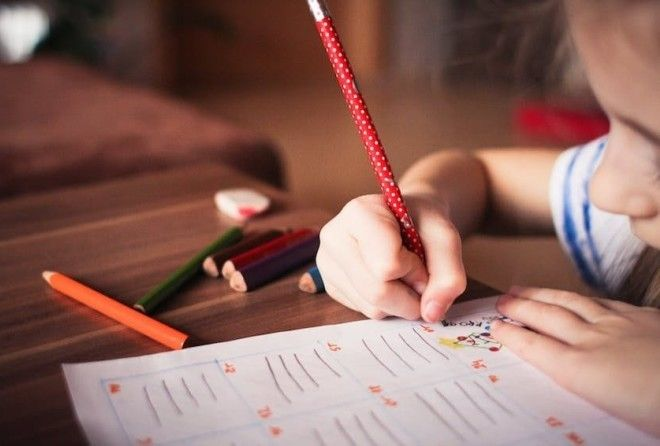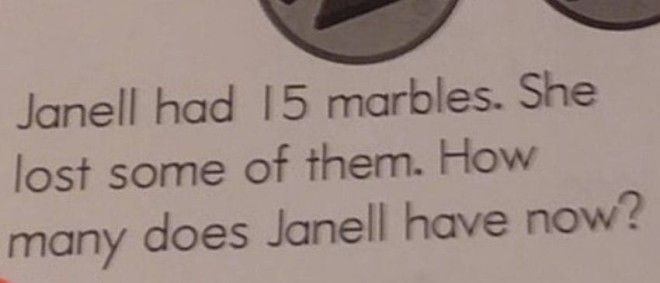Here is the notorious math problem.

It says, “Janell had 15 marbles. She lost some of them. How many does Janell have now?”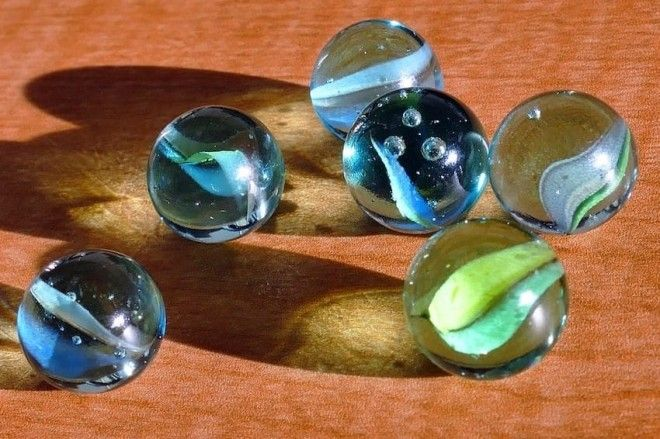If you’re thinking, “What?!” you’re not alone.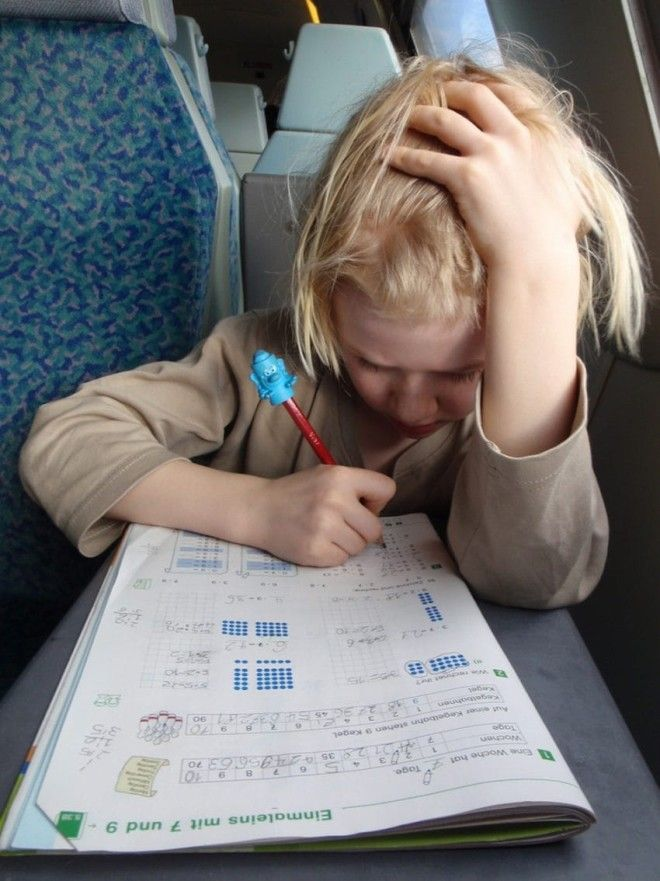Most people who saw this question were completely baffled by it.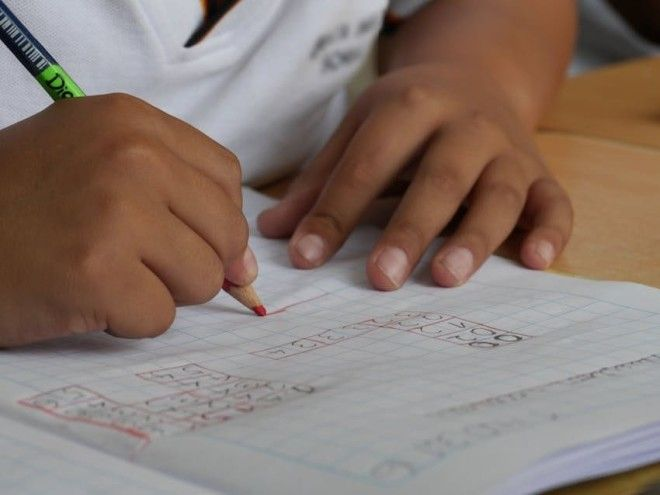So let’s take a look at some of the other math problems on the homework sheet to see if they’re just as confusing.

Here’s the full page of third-grade math homework.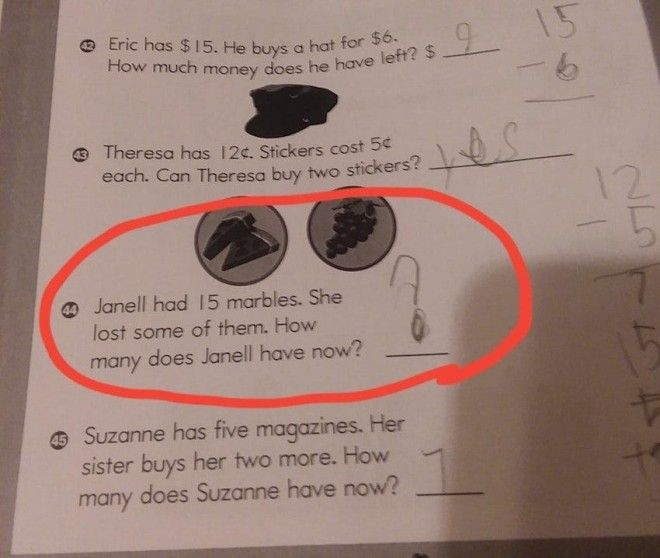Even the third grader seems frustrated by how confusing this problem is — she wrote in a giant question mark instead of an answer.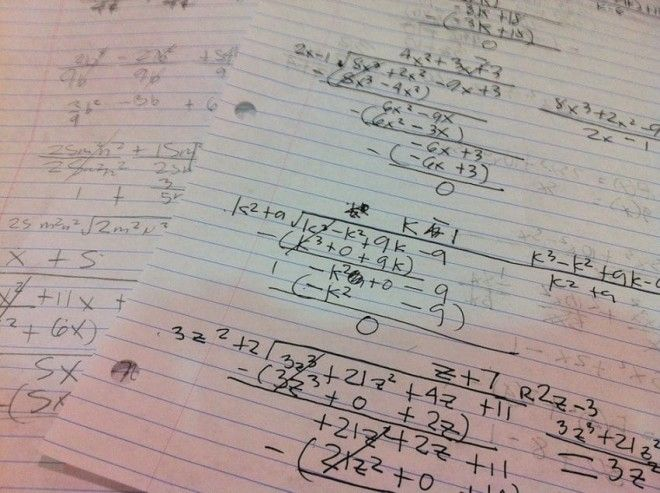The other questions are so simple and straightforward, which honestly makes this one all the more frustrating!

Let’s break this down logically to see if we can figure it out.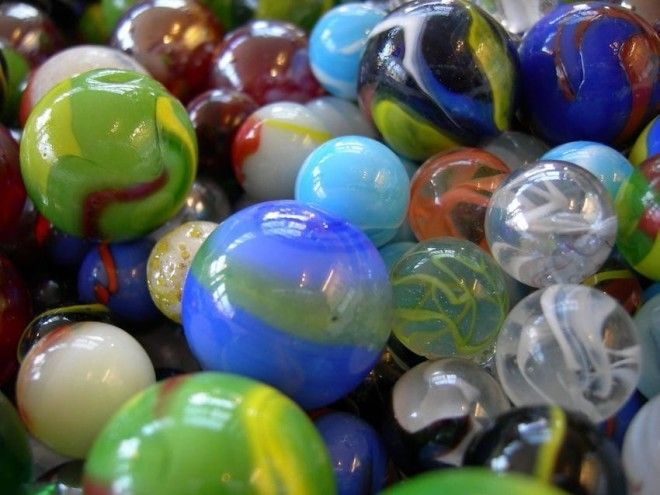OK, so Janell starts with 15 marbles. No questions there.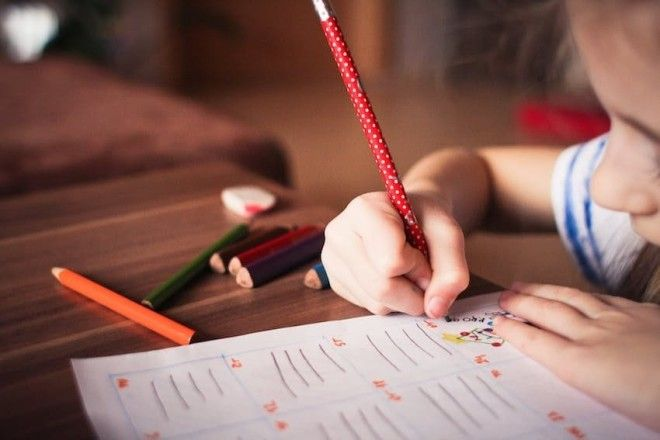The next part of the question says, “She lost some of them.”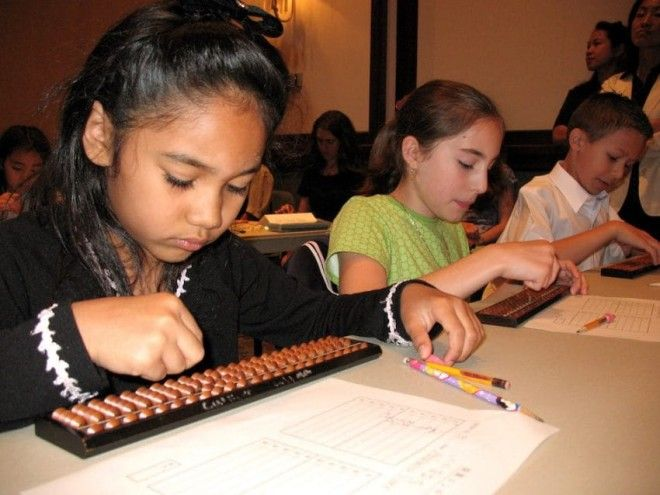This is where all the confusion comes from — how many is some?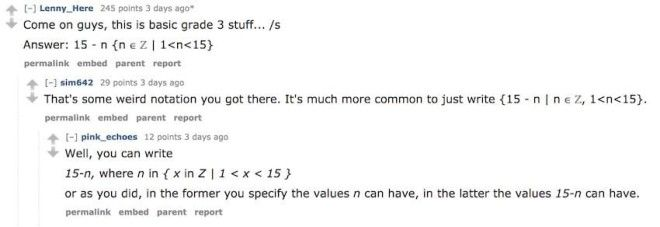According to the responses on Reddit, the answer is way more complicated than a third grader would understand.

Heck, it’s way more complicated than most college graduates would understand.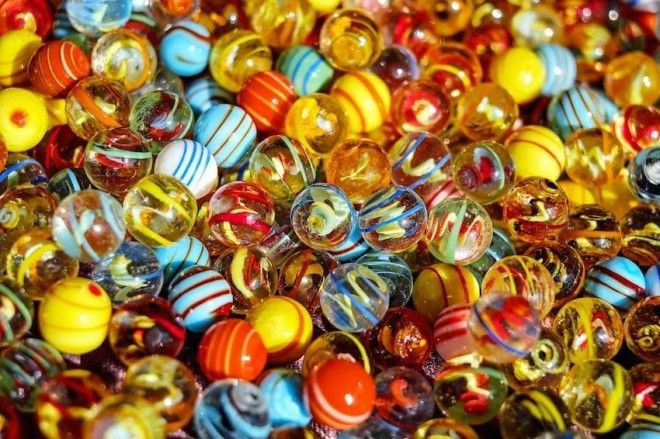In the simplest terms, the answer is “less than 15.”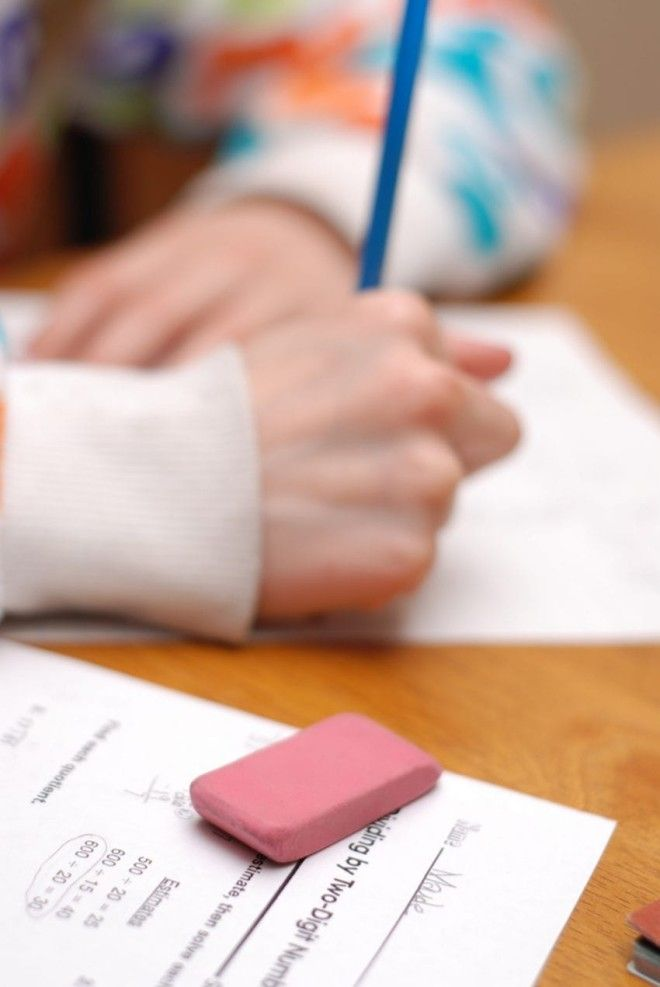No, that’s not an insanely difficult answer, but it’s definitely not nearly as straightforward as the rest of the problems on the assignment.According to one woman, the answer is even simpler: The teacher got distracted while writing the math problem.

Whether or not that’s true, we may never know, but that would certainly explain the issue.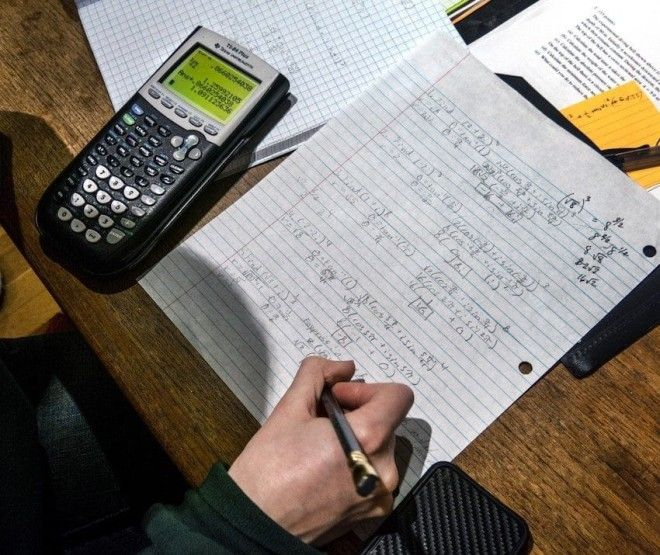Source